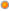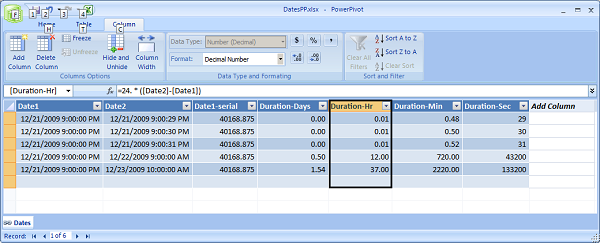# Q: How can I calculate difference between two dates in DAX (seconds, minutes, hours, days and months)

User Rating: 5 / 5Note: PowerPivot for Excel has a list of Time intelligence functions (example DatesBetween, DatesInPeriod) that can be used to calculated difference between dates. This post is NOT about these functions. There will be another post that will explain Time intelligence functions in more details.

### How can I calculate difference in days, hours, minutes and seconds between two dates in PowerPivot DAX

Microsoft Excel and PowerPivot internally stores dates and times as number representing the number of days since 1900-Jan-0, plus a fractional portion of a 24 hour day ddddd.tttttt. This number is called serial date-time. You can read more details about this here.

To see this serial date-time value you should multiple date value by 1.0 (or 1.). Lets say you have column Date1 with value "21/12/2009 21:00:00". If you will create PowerPivot formula "= 1. * [Date1]", then result of this new calculation will be "40168.88".

We can use this knowledge when calculating difference between 2 dates in PowerPivot for excel.

Lets say we have a table with 2 columns: Date1 and Date2. Here is sample data for our test (you can copy it to Excel and then create linked table):

 Date1 Date2 21/12/2009 21:00:00 21/12/2009 21:00:29 21/12/2009 21:00:00 21/12/2009 21:00:30 21/12/2009 21:00:00 21/12/2009 21:00:31 21/12/2009 21:00:00 22/12/2009 9:00:00 21/12/2009 21:00:00 23/12/2009 10:00:00

Now we can add calculated fields that will calculate duration in days, minutes, hours and days:

 Calculation Description Calculated column name DAX Formula Serial date-time value for field Date1 Date1-serial =1. * [Date1] Duration in days between Date2 and Date1 Duration-Days =1. * ([Date2]-[Date1]) Duration in hours between Date2 and Date1 Duration-Hours =24. * ([Date2]-[Date1]) Duration in minutes between Date2 and Date1 Duration-Min =24. * 60 * ([Date2]-[Date1]) Duration in seconds between Date2 and Date1 Duration-Sec =ROUND(24. * 60 * 60 * ([Date2]-[Date1]), 1)

As you probably noticed, for "Duration-Sec" calculation we used Round function as we wanted to see integers for Seconds. Most likely you will want to do the same type of rounding for your minute, hour and day calculations too.

When calculating difference for seconds, we subtract Date1 from Date2 and then we multiply result by 24 (because there are 24 hours in one day) then multiply by 60 (because there are 60 minutes in one hour) and then finally we multiply by 60 again (because there are 60 seconds in one minute). Similar logic applies to minute and hour calculation.

After adding these calculations to the PowerPivot, we get following results:

 Date1 Date2 Date1-serial Duration-Days Duration-Hours Duration-Min Duration-Sec 12/21/2009 9:00:00 PM 12/21/2009 9:00:29 PM 40168.875 0.00 0.01 0.48 29 12/21/2009 9:00:00 PM 12/21/2009 9:00:30 PM 40168.875 0.00 0.01 0.50 30 12/21/2009 9:00:00 PM 12/21/2009 9:00:31 PM 40168.875 0.00 0.01 0.52 31 12/21/2009 9:00:00 PM 12/22/2009 9:00:00 AM 40168.875 0.50 12.00 720.00 43200 12/21/2009 9:00:00 PM 12/23/2009 10:00:00 AM 40168.875 1.54 37.00 2220.00 133200

And here is screenshot of results after I applied some PowerPivot formatting:### How can I calculate difference in months between two dates in PowerPivot DAX

We will explain few different approaches on how to calculate number of months between 2 dates. Lets say we have 2 dates: Jan 29, 2009 and Feb 2, 2009. Both dates are in different months, even actual difference between them is 4 days.

"Round Up" option

For this option we do not use days in calculation - we count difference at the month level. Because first date is in January and second date is in February, we want our result to be 1. We can implement this type of calculation using following formula:

=(YEAR([Date2])-YEAR([Date1]))*12+MONTH([Date2])-MONTH([Date1])

"Round down" option

This method uses the number days in its calculations and rounds down to the nearest number of whole months. Difference between our sample dates would be 0. We can implement this type of calculation using following formula:

=IF(DAY([Date2])>=DAY([Date1]),0,-1)+(YEAR([Date2])-YEAR([Date1]) ) * 12 + MONTH([Date2]) - MONTH([Date1])

"30 day periods" options

This method counts how many 30 day periods are between 2 dates. You can round result as per your busines requirements. We can implement this type of calculation using following formula:

=ROUND(([Date2]-[Date1]) / 30, 1)

You can read more about "Round up" and "Round down" calculation options for Excel here.

Tags: dax, faq, excel

2007-2015 VidasSoft Systems Inc.